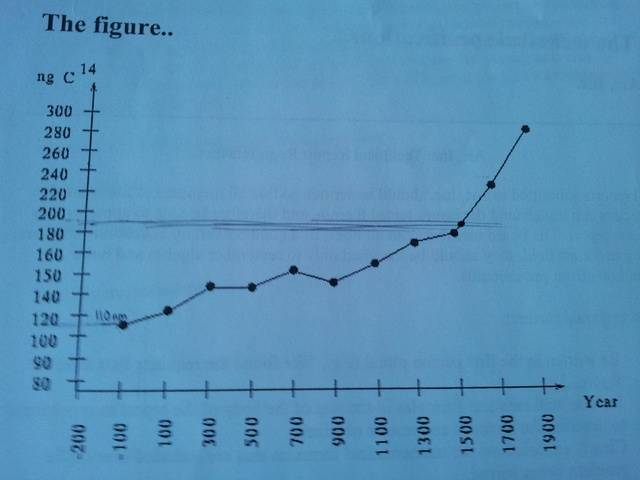# Carbon-14 Dating

## Homework Statement

An antiques dealer has claimed that a tapestry is 2000 years old having been loomed sometime in the first century BC. In order to determine the veracity of this claim a sample of Carbon-14 has been taken for dating. In a 1g sample, 190ng of Carbon-14 were present.

The half-life of Carbon-14 is 5780 years.
The below graph displays the amount of Carbon-14 present in the environment for the past 2100 years.Known variables:
Current Carbon-14 present: 190ng
Half life of Carbon-14: 5780 years

Wanted variables:
Age of tapestry (t)
And original amount of Carbon-14 ($N_{0}$)

## Homework Equations

Taken from Wikipedia.

$\frac{dN}{dt} = -\lambda N$
"...differential equation, where N is the number of radioactive atoms and λ is a positive number called the decay constant"

$N(t) = N_{0}e^{-\lambda t}$
"...describes an exponential decay over a timespan t with an initial condition of N0 radioactive atoms at t = 0. Canonically, t is 0 when the decay started. In this case, N0 is the initial number of 14C atoms when the decay started."

## The Attempt at a Solution

First I calculated the decay constant of C14.
$0.5 = e^{-\lambda 5780}$
$\lambda = 1.209681344 x 10^{-4}$

Since the current amount of C14 is 190ng, the sample cannot be older than ~1550, since there was ~190ng of C14 in the environment at that time.

However, this leaves me with two unknowns, $N_{0}$ and $t$.

If I try to get the original amount of C14:
$N(0) = N_{0}e^{-\lambda 0} = N_{0}e^{0} = N_{0}(1)$
$N(0) = N_{0}$???

We've never done Carbon-14 dating before, and everything I've found so far has been from my own research. I feel as though I'm not understanding this correctly, and I'm unsure how to go about solving this.
Any and all guidance would be greatly appreciated.

Last edited:

look your lamda (decay constant) is equal to -(ln2/half life ( in years)) and then N(t)=Ninitial (which is the amount you started with) x e^(lamda x t (time in years))..
Hope this helped

I have since figured this out.
For those who may want to reference this in the future, here's what I did.
I took the graph of environmental C-14 and linearized it into an equation from the year 1500 to 1900.
Since the current-day value of C-14 is only 190ng and the environmental levels are no higher than that until 1500 I could rule out all previous years.
Then, using my function for the environmental levels of C-14 I graphed it and found the intersection with the graph of the exponential decay of C-14.
My linearized function:
$C(t) = 0.265(x-450) + 180$
The exponential decay of C-14 solved for $N_{0}$:
$N_{0}(t) = \frac{190}{e^{-\lambda t}}$
Then I graphed them and found the intersection, then calculated the year from the resulting data.
The year I got was approximately 1568.

hunt_mat
Homework Helper
You can do this using calculus. The thing which you glossed over was the solution of the differential equation.
$$\frac{dN}{dt}=-\lambda N$$
Integrating this equation you obtain:
$$\ln N=-\lambda t+C$$
Not to compute $C$, what you did was automatically did was set t=0, to show that $C=\ln N(0)=\ln N_{0}$, you are however free to choose the present time if you wish, which is what you should have done. From there you could have calculated the time from the amount you had today.

The below graph displays the amount of Carbon-14 present in the environment for the past 2100 years....

..We've never done Carbon-14 dating before, and everything I've found so far has been from my own research.

That is admirable, but a few thoughts on this (but only from a maths/statistics/common-sense perspective, not an 'expert' one).

Any graph showing values should be scrutinised to see if it makes sense, before using it. We need to understand what that graph you have shows. With a half-life of >5,000 years, I'd not expect the variation from 1500AD back to -100BC as showing a reduction of a half.

Similarly, I would not expect to see a function of 14C over time to show only reductions. I would expect to see statistical 'bouncing around' a trend. I suppose that is possible in this graph, but doesn't look distinctly to be the case, with it shooting up suddenly at 1500AD.

So I would tend to conclude this as meaning it is a composite graph of both the exponential decay of 14C, together with some form of calibration result that normalises the results for use today.

If that interpretation is correct, then you need not be looking at exponential rates, as the graph does it for you and I'm guessing this graphs shows, quite simply, that you draw your line, as has been done, at the measured value and you read off the answer.

I'm not suggesting you take that as an answer, but merely to say you should think through whether the information you have, and the interpretation of it you have arrived at, makes sense., because to me it looks suspect that this graph is of what was 'present', more like 'what is left of...'.

epenguin
Homework Helper
Gold Member
For a crude estimate and discrimination needed by an antiques dealer i,e, whether the sample is ancient or modern or even a medieval forgery etc. just a half-life calculation would be sufficient. If however the exact dates are required there are refined 'calibrations', essentially the atmospheric levels of C14 over recent millennia are known though there can be disputes and revisions among experts. Due to this in lucky cases - when the level was varying fast - a sample can be radiocarbon dated to even as little as five years, at worst it is 50 years with a homogeneous sample.However I question what that diagram of the OP represents. It is far too fast to represent C14 against age, and it seems to me far too big and monotonic to represent C14 content of atmosphere at those times.